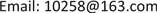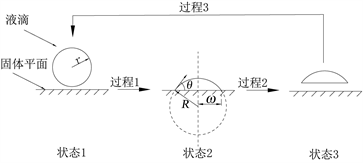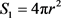﻿ 液滴铺展过程的能量转换 The Energy Conversion during the Droplet Spreading

International Journal of Mechanics Research
Vol. 07  No. 04 ( 2018 ), Article ID: 27852 , 5 pages
10.12677/IJM.2018.74014

The Energy Conversion during the Droplet Spreading

Yuan Wen

Zhejiang Rotoun Plastic Technology Co., Ltd., Ningbo ZhejiangReceived: Nov. 14th, 2018; accepted: Nov. 27th, 2018; published: Dec. 4th, 2018ABSTRACT

The prevailing view considers that the surface energy has been involved in viscosity loss during the droplet spreading. But analyzed theoretically by spherical deficiency hypothetical model, the result shows the system internal energy conservation during the spreading (except the molecular kinetic energy), free of losses, and that surface energy translates into adhesional energy and deformation energy (molecular potential energy). The energy resource of viscous dissipation is only possible the system mechanical energy. During the droplet spreading, the acting of horizontal component of surface tension leads to the conversion of system internal energy, the acting of vertical component of surface tension participates into the viscous dissipation process.

Keywords:Viscous Dissipation, Energy, Surface Tension, Droplet Spreading1. 引言

2. 分析模型Figure 1. Hypothetical process schematic diagram(1)

${V}_{1}=\frac{4}{3}\text{π}{r}^{3}$ (2)

${E}_{B1}={E}_{S1}+{E}_{L1}={\gamma }_{S}{A}_{1}+{\gamma }_{L}{S}_{1}$ (3)

${S}_{2}=2\text{π}{R}^{2}\left(1-\mathrm{cos}\theta \right)$ (4)

${V}_{2}=\frac{1}{3}\text{π}{R}^{3}{\left(1-\mathrm{cos}\theta \right)}^{2}\left(2+\mathrm{cos}\theta \right)$ (5)

${A}_{2}=\text{π}{\omega }^{2}=\text{π}{R}^{2}{\mathrm{sin}}^{2}\theta$ (6)

${E}_{B2}={E}_{S2}+{E}_{L2}+{E}_{LS2}={\gamma }_{S}\left({A}_{1}-{A}_{2}\right)+{\gamma }_{L}{S}_{2}+{\gamma }_{LS}{A}_{2}$ (7)

${W}_{N}=\left({\gamma }_{L}+{\gamma }_{S}-{\gamma }_{SL}\right){A}_{2}$ (8)

$\frac{4{r}^{3}}{{R}^{3}}={\left(1-\mathrm{cos}\theta \right)}^{2}\left(2+\mathrm{cos}\theta \right)$ (9)

${E}_{B3}={E}_{S3}+{E}_{L3}={\gamma }_{S}{A}_{1}+{\gamma }_{L}\left({S}_{2}+{A}_{2}\right)$ (10)

${E}_{X3}-{E}_{X1}={E}_{B1}-{E}_{B3}={\gamma }_{L}\left({S}_{1}-{S}_{2}-{A}_{2}\right)$ (11)

3. 表面能

${E}_{B1}+{E}_{X1}={E}_{B2}+{E}_{X2}+{E}_{N}$ (12)

${W}_{B1}={\int }_{0}^{{A}_{2}}\left({\gamma }_{S}-{\gamma }_{SL}-{\gamma }_{L}\mathrm{cos}\theta \right)\text{d}{A}_{2}$ (13)

${\gamma }_{L}\mathrm{cos}{\theta }_{P}={\gamma }_{S}-{\gamma }_{SL}$ (14)

${W}_{B1}=\left[1-\frac{2-\mathrm{cos}{\theta }_{P}\left(1+\mathrm{cos}\theta \right)}{\sqrt{4\left(1-\mathrm{cos}\theta \right){\left(2+\mathrm{cos}\theta \right)}^{2}}}\right]{E}_{L1}=-\left({E}_{B2}-{E}_{B1}\right)$ (15)

${W}_{B1}=\left(1-\frac{r}{{R}_{p}}\right){E}_{L1}$ (16)

$F={\gamma }_{S}-{\gamma }_{SL}-{\gamma }_{L}\mathrm{cos}\theta$ (16a)

4. 压力能

${E}_{Y1}=\int \text{d}\left({P}_{1}{V}_{1}\right)=\frac{2{\gamma }_{L}}{r}×\frac{4}{3}\text{π}{r}^{3}=\frac{2}{3}{E}_{L1}$ (17)

${E}_{Y2}=\int \text{d}\left({P}_{2}{V}_{1}\right)=\frac{2{\gamma }_{L}}{R}×\frac{4}{3}\text{π}{r}^{3}=\frac{2r}{3R}{E}_{L1}$ (18)

${W}_{B2}=-\left({E}_{Y2}-{E}_{Y1}\right)=\frac{2}{3}\left(1-\frac{r}{R}\right){E}_{L1}$ (19)

${W}_{B}={W}_{B1}+{W}_{B2}=\frac{5}{3}\left(1-\frac{r}{{R}_{P}}\right){E}_{L1}$ (20)

5. 粘性损耗

${W}_{\varphi }={W}_{B2}=\frac{2}{3}\left(1-\frac{r}{R}\right){E}_{L1}$ (21)

${W}_{\varphi }=\Delta {E}_{动能}+\Delta {E}_{表面能}$ (22)

6. 结论

1) 液滴铺展过程中，内能守恒，表面能、粘附能和变形能相互转化，没有参与粘性耗散；

2) 粘性耗散的主要能量来源于压力能；

3) 表面张力的水平分量做功引起了内能的转化，垂直分量做功引起了粘性耗散。

The Energy Conversion during the Droplet Spreading[J]. 力学研究, 2018, 07(04): 121-125. https://doi.org/10.12677/IJM.2018.74014

1. 1. Chandra, S. and Avedisian, C.T. (1991) On the Collision of a Droplet with a Solid Surface. Proceedings: Mathematical and Physical Sciences, 432, 13-41.

2. 2. 施明恒. 单个液滴碰击表面时的流体动力学特性[J]. 力学学报, 1985, 17(5): 419-425.

3. 3. 廖强, 邢淑敏, 朱恂, 王宏. 水平均质表面上非等径水滴聚合过程的可视化实验[J]. 热科学与技术, 2006, 5(3): 211-215.

4. 4. 秦梦晓, 张旭辉, 汤成龙. 液滴撞击不同粗糙度固体表面动力学行为实验研究[J]. 西安交通大学学报, 2017, 51(9): 26-31.

5. 5. 王辉. 液滴碰撞壁面振荡的特性分析[D]: [硕士学位论文]. 大连: 大连理工大学, 2013.

6. 6. 崔正刚. 表面活性剂、胶体与界面化学基础[M]. 北京: 化学工业出版社, 2013: 11-12.

7. 7. 陶英. 平壁液滴静态铺展的理论研究[D]: [硕士学位论文]. 大连: 大连理工大学, 2014.

8. 8. 李大树, 仇性启, 于磊, 等. 柴油液滴冲击液膜润湿壁面实验和数值模[J]. 农业机械学报, 2014, 45(12): 9-17.

9. 9. 李德伟. 液滴碰壁铺展与振荡的研究[D]: [硕士学位论文]. 大连: 大连理工大学, 2015.

10. 10. 马淑清, 王鉴, 胡烨. 拉普拉斯方程不同推导方法及其意义[J]. 教育教学论坛, 2014(2): 97-98.

11. 11. 郑志伟, 李大树, 仇性启, 等. 中空液滴碰撞水平壁面数值分析[J]. 物理学报, 2017, 66(1): 235-244.

12. 12. 李西营. 液滴撞击固体壁面的实验及理论研究[D]: [博士学位论文]. 大连: 大连理工大学, 2010.